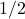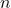# Learning Theory: Purely Theoretical?

What’s learning theory good for, anyway? As I mentioned in my earlier blog post, not infrequently get into conversations with people in machine learning and related fields who don’t see the benefit of learning theory (that is, theory of learning). While that post offered one specific piece of evidence of how work seemingly only relevant in pure theory could lead to practical algorithms, I thought I would talk in more general terms why I see learning theory as a worthwhile endeavor.

There are two main flavors of learning theory, statistical learning theory (StatLT) and computational learning (CompLT). StatLT originated with Vladimir Vapnik, while the canonical example of CompLT, PAC learning, was formulated by Leslie Valiant. StatLT, in line with its “statistical” descriptor, focuses on asymptotic questions (though generally based on useful non-asymptotic bounds). It is less concerned with computational efficiency, which is where CompLT comes in. Computer scientists are all about efficient algorithms (which for the purposes of theory essentially means polynomial vs. super-polynomial time). Generally, StatLT results apply to a wider variety of hypothesis classes, with few or no assumptions made about the concept class (a concept class refers to the class of functions to which the data generating mechanism belongs). CompLT results apply to very specific concept classes but have stronger performance guarantees, often using polynomial time algorithms. I’ll do my best to defend both flavors, while also mentioning some of their limitations.

So, what is learning theory good for? I think there are both practical and philosophical reasons, four of which I will discuss below. At the end, I’ll mention some of the major areas where existing theories fall short.

1. The occasional very practical algorithm. The regret/risk bounds and algorithms derived in the pursuit of  (or based on) theoretical guarantees can lead to practical algorithms. In the case of StatLT, there is Vapnik’s structural risk minimization (SRM) framework, which provides generalization guarantees in terms of risk bounds. SRM led to the development of support vector machines, which have proven very useful in practice, independent of their theoretical properties. SVMs are a popular out-of-the-box classifier option since they require minimal tuning and, with the proper precautions, work quite well on most data sets. Hence, for many practitioners,  the cost of searching out the optimal classifier solution greatly outweighs the marginal improvement in performance that method would provide over SVMs. As for CompLT, the question of turning “weak learners” (learners that classify better than random chance) into “strong learners” (learners that can classify with arbitrarily small probability of error, given enough data) led to boosting methods, with AdaBoost (due to Freund and Schapire) being of particular note.  Other interpretations of AdaBoost have been developed which make it relevant even for algorithms that aren’t “weak learners.” For example, in an StatLT framework, boosting can also be viewed as finding the risk minimizer for a exponential loss from among a convex set of functions (a result due to Zhang [pdf]). I think an argument could be made the SVMs and AdaBoost alone justify the existence of learning theory. But I don’t need defend such a bold statement, since I still have three more points discuss!

2. Formalizing what is “learnable.” This argument is analogous to one of the arguments for studying complexity theory. Namely, that it’s important to know which problems can’t be solved efficiently. Think of all the wasted effort if researchers were spending valuable time trying to find a polynomial time algorithm for traveling salesman. Until the incredibly unlikely that a theorist comes along and proves P = NP, the world is quite content to find approximation algorithms or solve special cases. Analogously, results on the hardness of learning tell us which classes of functions are (likely to be) difficult to learn. For example,-sized boolean formulas can’t be PAC-learned intime. Such a result esnures we should either (a) give up on learning-sized boolean formulas or (b) consider an alternative framework with a looser definition of what it means to learn. Thus, these results help guide our thinking about what can be realistically learned and what we even mean by learning.

3. Intuition about what learning rates we can hope to achieve. This argument has a similar flavor to #2, but I separate it out because whereas #2 is about theorems and specific results, this is about gaining conceptual insight that is independent of a particular problem. For example, in both StatLT and CompLT, results are usually phrased as holding “with high probability” (i.e. with probability greater than). Both theories show that the sample complexity “should” scale like. There’s a very simple, general argument for why this should be, but nonetheless it’s worth keeping in mind because it tells us that our main concern shouldn’t be what happens if we get unlucky (with unrepresentative data). We can make the probability of that exponentially small at only a polynomial cost. Our real concern should be our “error” parameter, call it, for which sample complexity scales like. There’s good reason to think this should hold –– we can’t expect the amount we learn to increase exponentially with the amount of data. Specific theories and situations can provide more fine-grained intuition. For example, StatLT tells us risk/error bounds usually decrease like. Such understanding can provide realistic expectations of what might happen if, say, we double the amount of labeled data we have (in this case, we should expect only afactor decrease in “”, not a).

4. Controlling model complexity and Occam’s razor-like results. Many theorems in CompLT and StatLT provide instantiations of Occam’s razor: “The simpler explanation is to be preferred.”* The simplest version of this, from PAC learning, is known as “Occam’s razor theorem.” Roughly speaking, it states we don’t want to choose from too many hypotheses compared to the amount of data we have. In particular, we want the size of the hypothesis space used to grow like, whereis the number of data points we are trying to explain. Note that there arepossible boolean functions onbinary variables, so exponential growth is actually slow. The myriad results in both CompLT and StatLT that rely on various measures of the complexity of hypothesis classes — such as VC dimension, fat-shattering dimension, Rademacher and Gaussian complexity, and covering numbers — generalize the simple PAC Occam’s razor result to realistic scenarios in which the hypothesis class has uncountably many functions. These complexity measures have many close connections with each other. Combined the risk bounds and lower bounds on learning rates they appear in, they can help us to understand and compare different hypothesis classes for which size and number of parameters are not good measures of complexity. A canonical example of the number of parameters being a bad measure of complexity is that the sine function (1 parameter) has infinite VC dimension while a-dimensional hyperplane (parameters) has VC dimension. A practical example where complexity measures  provide important insight come from kernel learning methods, which project data into an infinite-dimensional space. The fact that such methods work would seem to suggest they are magically avoiding the “curse of dimensionality.” But by looking at complexity measures for specific kernels, one finds that their effective dimensionality is very much finite. As a representative example, Zhang (2005) [pdf] shows that the effective dimension for kernel regression with an exponential kernel is, whereis the number of data points.

So there you go. I hope I’ve convinced the unbelievers and skeptics that learning theory does in fact offer significant value to those interested in practical learning question. Clearly, it is not a panacea and fails to capture important aspects of real-world learning problems. There are many algorithms that work well in practice for which we have no theoretical justification. But, as far as I’m concerned, such shortcomings are good justification for continuing to work on developing better theory.

*In the interest of completeness, I should mention that outside CompLT and StatLT, there’s also the “Bayesian Occam’s razor” theorem that gets at the same idea for Bayesian inference.